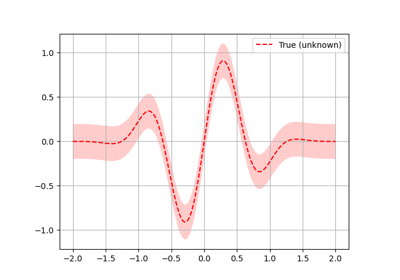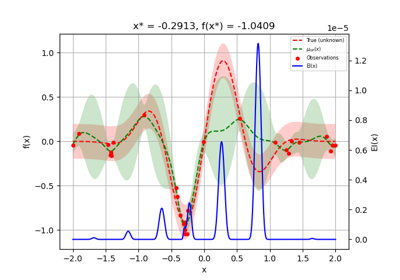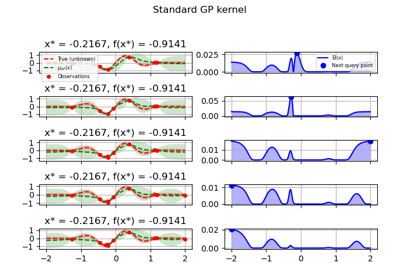# skopt.Optimizer¶

class skopt.Optimizer(dimensions, base_estimator='gp', n_random_starts=None, n_initial_points=10, initial_point_generator='random', n_jobs=1, acq_func='gp_hedge', acq_optimizer='auto', random_state=None, model_queue_size=None, acq_func_kwargs=None, acq_optimizer_kwargs=None)[source][source]

Run bayesian optimisation loop.

An Optimizer represents the steps of a bayesian optimisation loop. To use it you need to provide your own loop mechanism. The various optimisers provided by skopt use this class under the hood.

Use this class directly if you want to control the iterations of your bayesian optimisation loop.

Parameters
dimensionslist, shape (n_dims,)

List of search space dimensions. Each search dimension can be defined either as

• a (lower_bound, upper_bound) tuple (for Real or Integer dimensions),

• a (lower_bound, upper_bound, "prior") tuple (for Real dimensions),

• as a list of categories (for Categorical dimensions), or

• an instance of a Dimension object (Real, Integer or Categorical).

base_estimator"GP", "RF", "ET", "GBRT" or sklearn regressor, default: "GP"

Should inherit from sklearn.base.RegressorMixin. In addition the predict method, should have an optional return_std argument, which returns std(Y | x) along with E[Y | x]. If base_estimator is one of [“GP”, “RF”, “ET”, “GBRT”], a default surrogate model of the corresponding type is used corresponding to what is used in the minimize functions.

n_random_startsint, default: 10

Deprecated since version 0.6: use n_initial_points instead.

n_initial_pointsint, default: 10

Number of evaluations of func with initialization points before approximating it with base_estimator. Initial point generator can be changed by setting initial_point_generator.

initial_point_generatorstr, InitialPointGenerator instance, default: "random"

Sets a initial points generator. Can be either

• "random" for uniform random numbers,

• "sobol" for a Sobol sequence,

• "halton" for a Halton sequence,

• "hammersly" for a Hammersly sequence,

• "lhs" for a latin hypercube sequence,

• "grid" for a uniform grid sequence

acq_funcstring, default: "gp_hedge"

Function to minimize over the posterior distribution. Can be either

• "LCB" for lower confidence bound.

• "EI" for negative expected improvement.

• "PI" for negative probability of improvement.

• "gp_hedge" Probabilistically choose one of the above three acquisition functions at every iteration.

• The gains g_i are initialized to zero.

• At every iteration,

• Each acquisition function is optimised independently to propose an candidate point X_i.

• Out of all these candidate points, the next point X_best is chosen by $$softmax(\eta g_i)$$

• After fitting the surrogate model with (X_best, y_best), the gains are updated such that $$g_i -= \mu(X_i)$$

• "EIps" for negated expected improvement per second to take into account the function compute time. Then, the objective function is assumed to return two values, the first being the objective value and the second being the time taken in seconds.

• "PIps" for negated probability of improvement per second. The return type of the objective function is assumed to be similar to that of "EIps"

acq_optimizerstring, "sampling" or "lbfgs", default: "auto"

Method to minimize the acquisition function. The fit model is updated with the optimal value obtained by optimizing acq_func with acq_optimizer.

• If set to "auto", then acq_optimizer is configured on the basis of the base_estimator and the space searched over. If the space is Categorical or if the estimator provided based on tree-models then this is set to be "sampling".

• If set to "sampling", then acq_func is optimized by computing acq_func at n_points randomly sampled points.

• If set to "lbfgs", then acq_func is optimized by

• Sampling n_restarts_optimizer points randomly.

• "lbfgs" is run for 20 iterations with these points as initial points to find local minima.

• The optimal of these local minima is used to update the prior.

random_stateint, RandomState instance, or None (default)

Set random state to something other than None for reproducible results.

n_jobsint, default: 1

The number of jobs to run in parallel in the base_estimator, if the base_estimator supports n_jobs as parameter and base_estimator was given as string. If -1, then the number of jobs is set to the number of cores.

acq_func_kwargsdict

Additional arguments to be passed to the acquisition function.

acq_optimizer_kwargsdict

Additional arguments to be passed to the acquisition optimizer.

model_queue_sizeint or None, default: None

Keeps list of models only as long as the argument given. In the case of None, the list has no capped length.

Attributes
Xilist

Points at which objective has been evaluated.

yiscalar

Values of objective at corresponding points in Xi.

modelslist

Regression models used to fit observations and compute acquisition function.

spaceSpace

An instance of skopt.space.Space. Stores parameter search space used to sample points, bounds, and type of parameters.

Methods

 ask([n_points, strategy]) Query point or multiple points at which objective should be evaluated. copy([random_state]) Create a shallow copy of an instance of the optimizer. Returns the same result that would be returned by opt.tell() but without calling tell run(func[, n_iter]) Execute ask() + tell() n_iter times tell(x, y[, fit]) Record an observation (or several) of the objective function. Updates the value returned by opt.ask().
__init__(dimensions, base_estimator='gp', n_random_starts=None, n_initial_points=10, initial_point_generator='random', n_jobs=1, acq_func='gp_hedge', acq_optimizer='auto', random_state=None, model_queue_size=None, acq_func_kwargs=None, acq_optimizer_kwargs=None)[source][source]

Initialize self. See help(type(self)) for accurate signature.

ask(n_points=None, strategy='cl_min')[source][source]

Query point or multiple points at which objective should be evaluated.

n_pointsint or None, default: None

Number of points returned by the ask method. If the value is None, a single point to evaluate is returned. Otherwise a list of points to evaluate is returned of size n_points. This is useful if you can evaluate your objective in parallel, and thus obtain more objective function evaluations per unit of time.

strategystring, default: “cl_min”

Method to use to sample multiple points (see also n_points description). This parameter is ignored if n_points = None. Supported options are "cl_min", "cl_mean" or "cl_max".

• If set to "cl_min", then constant liar strategy is used

with lie objective value being minimum of observed objective values. "cl_mean" and "cl_max" means mean and max of values respectively. For details on this strategy see:

https://hal.archives-ouvertes.fr/hal-00732512/document

With this strategy a copy of optimizer is created, which is then asked for a point, and the point is told to the copy of optimizer with some fake objective (lie), the next point is asked from copy, it is also told to the copy with fake objective and so on. The type of lie defines different flavours of cl_x strategies.

copy(random_state=None)[source][source]

Create a shallow copy of an instance of the optimizer.

Parameters
random_stateint, RandomState instance, or None (default)

Set the random state of the copy.

get_result()[source][source]

Returns the same result that would be returned by opt.tell() but without calling tell

Returns
resOptimizeResult, scipy object

OptimizeResult instance with the required information.

run(func, n_iter=1)[source][source]

Execute ask() + tell() n_iter times

tell(x, y, fit=True)[source][source]

Record an observation (or several) of the objective function.

Provide values of the objective function at points suggested by ask() or other points. By default a new model will be fit to all observations. The new model is used to suggest the next point at which to evaluate the objective. This point can be retrieved by calling ask().

To add observations without fitting a new model set fit to False.

To add multiple observations in a batch pass a list-of-lists for x and a list of scalars for y.

Parameters
xlist or list-of-lists

Point at which objective was evaluated.

yscalar or list

Value of objective at x.

fitbool, default: True

Fit a model to observed evaluations of the objective. A model will only be fitted after n_initial_points points have been told to the optimizer irrespective of the value of fit.

update_next()[source][source]

## Examples using skopt.Optimizer¶